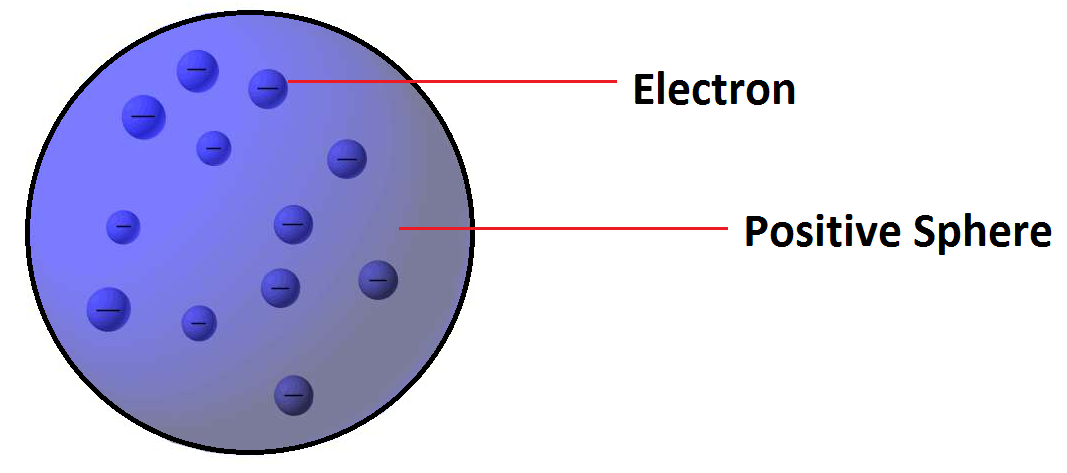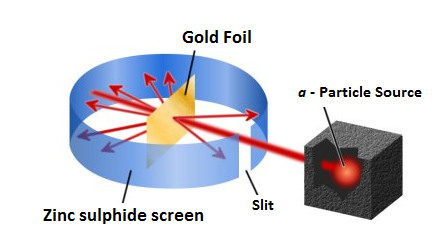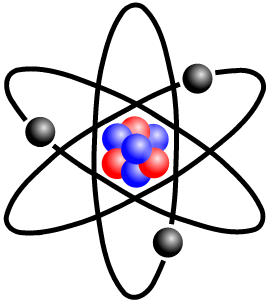×#### Thank you for registering.

One of our academic counsellors will contact you within 1 working day.

Click to Chat

1800-1023-196

+91-120-4616500

CART 0

• 0

MY CART (5)

Use Coupon: CART20 and get 20% off on all online Study Material

ITEM
DETAILS
MRP
DISCOUNT
FINAL PRICE
Total Price: Rs.

There are no items in this cart.
Continue ShoppingAtomic Models

Table of Content

Thomson’s Model

Rutherford’s Model

Gold Foil Experiment

Observation of Gold Foil Experiment

Conclusions of Rutherford by Gold Foil Experiment

Atomic Model

Defects of Rutherford’s Atomic Model

Bohr’s Atomic Model

Important Postulates of Niels Bohr Atomic Theory

Calculation of Radius and Energy Levels of Hydrogen Atom

Calculation of energy of an electron

Calculation of velocity of Electron

Merits of Bohr’s theory

Limitations of Bohr’s theoryWe know the fundamental particles of the atom. Now let us see, how these particles are arranged in an atom to suggest a model of the atom.

Thomson’s Model

J.J. Thomson, in 1904, presented the first atomic model. He proposed that an atom was a sphere of positive electricity in which were embedded a number of electrons, sufficient to neutralize the positive charge. The stability of the atom was explained as a result of the balance between the repulsive forces between the electrons and their attraction towards the centre of the positive sphere.This model could not satisfactorily explain the results of scattering experiment carried out by Rutherford who worked with Thomson.

Rutherford’s Model

Refer to the following video for Rutherford’d gold foil experiment and atomic model

Gold Foil Experiment

α– particles emitted by radioactive substance were shown to be dipositive Helium ions (He++) having a mass of 4 units and 2 units of positive charge.

Rutherford allowed a narrow beam of α–particles to fall on a very thin gold foil of thickness of the order of 0.0004 cm and determined the subsequent path of these particles with the help of a zinc sulphide fluorescent screen. The zinc sulphide screen gives off a visible flash of light when struck by  a particle, as ZnS has the remarkable property of converting kinetic energy of α particle into visible light. [For this experiment, Rutherford specifically used α particles because they are relatively heavy resulting in high momentum].Observation of Gold Foil Experiment:

1. Majority of the a–particles pass straight through the gold strip with little or no deflection.

2. Some α–particles are deflected from their path and diverge.

3. Very few α–particles are deflected backwards through angles greater than 90°.

4. Some were even scattered in the opposite direction at an angle of 180°[Rutherford was very much surprised by it and remarked that “It was as incredible as if you fired a 15­–inch shell at a piece of tissue paper and it came back and hit you”.

There is far less difference between air and bullet than there is between gold atoms and α-particle assuming of course that density of a gold atom is evenly distributed. The distance of nucleus from where the α - particle returns back through 180° is called distance of closet approach and is given by  ro = q1q2 / 4πεo ( 1/2mv2)

Conclusions of Rutherford by Gold Foil Experiment:

1. The fact that most of the α - particles passed straight through the metal foil indicates the most part of the atom is empty.

2. The fact that few α - particles are deflected at large angles indicates the presence of a heavy positively charge body i.e., for such large deflections to occur α - particles must have come closer to or collided with a massive positively charged body.

3. The fact that one in 20,000 have deflected at 180° backwards indicates that volume occupied by this heavy positively charged body is very small in comparison to total volume of the atom.

Atomic Model

1. On the basis of the above observation, Rutherford proposed an atomic model as follows.

2. All the protons (+ve charge) and the neutrons (neutral charge) i.e nearly the total mass of an atom is present in a very small region at the centre of the atom. The atom’s central core is called nucleus.

3. The size of the nucleus is very small in comparison to the size of the atom. Diameter of the nucleus is about 10–13cm while the atom has a diameter of the order of 10–8 cm. So, the size of atom is 105 times more than that of nucleus.

4. Most of the space outside the nucleus is empty.

5. The electrons, equal in number to the net nuclear positive charge, revolve around the nucleus with fast speed in various circular orbits.

6. The centrifugal force  arising due to the fast speed of an electron balances the coulombic force of attraction of the nucleus and the electron remains stable in its path. Thus according to him atom consists of two parts (a) nucleus and (b) extra nuclear part.

Defects of Rutherford’s Atomic Model

1. Position of electrons:  The exact positions of the electrons from the nucleus are not mentioned.

2. Stability of the atom: Neils Bohr pointed out that Rutherford’s atom should be highly unstable. According to the law of electro–dynamics, the electron should therefore, continuously emit radiation and loseenergy. As a result of this a moving electron will come closer and closer  to the nucleus and after passing through a spiral path, it should ultimately fall into the nucleus. It was calculated that the electron should fall into the nucleus in less than 10–8 sec.
But it is known that electrons keep moving outside the nucleus. To solve this problem Neils Bohr proposed an improved form of Rutherford’s atomic model. Before going into the details of Neils Bohr model we would like to introduce you some important atomic terms.???

Atomic Term

Explanation

Nuclide

Various species of atoms in general.

Nucleons

Sub-atomic particles in the nucleus of an atom, i.e., protons and neutrons.

Isotopes

Atoms of an element with the same atomic number but different mass number.

Mass number (A)

Sum of the number of protons and neutrons, i.e., the total number of nucleons

Atomic number (Z)

The number of protons in the nucleus of an atom. This, when subtracted from A, gives the number of neutrons.

Isobars

Atoms, having the same mass numbers but different atomic numbers, e.g., 15P32 and16S32.

Isotones

Atoms having the same number of neutrons but different number of protons or mass number, e.g., 146C, 157N.

Isoelectronic species

Atoms molecules or ions having the same number of electrons, e.g., N2, CO, CN-.

Nuclear isomers

Atoms with the same atomic and mass numbers but different radioactive properties, e.g., uranium X (half life 1.4 min) and uranium Z (half life 6.7 hours).

Atomic mass unit

Exactly equal to 1/12th of the mass of 6C12 atom.

(a.m.u.): 1 a.m.u. = 1.66 x10–24 g ≈ 931.5 MeV

Bohr’s Atomic Model

Refer the following video for Bohr’s Atomic ModelBohr developed a model for hydrogen atom and hydrogen like one–electron species (hydrogenic species). He applied quantum theory in considering the energy of an electron bound to the nucleus.

Important Postulates of Niels Bohr Atomic Theory:

1. An atom consists of a dense nucleus situated at the centre with the electron revolving around it in circular orbits without emitting any energy. The force of attraction between the nucleus and an electron is equal to the centrifugal force of the moving electron.

2. Of the finite number of circular orbits around the nucleus, an electron can revolve only in those orbits whose angular momentum (mvr) is an integral multiple of factor h/2π .

Where, m = mass of the electron

v = velocity of the electron

n = orbit number in which electron is present

r = radius of the orbit

1. As long as an electron is revolving in an orbit it neither loses nor gains energy. Hence these orbits are called stationary states. Each stationary state is associated with a definite amount of energy and it is also known as energy levels. The greater the distance of the energy level from the nucleus, the more is the energy associated with it. The different energy levels are numbered as 1,2,3,4, (from nucleus onwards) or K, L, M, N etc.

2. Ordinarily an electron continues to move in a particular stationary state without losing energy. Such a stable state of the atom is called as ground state or normal state.

3. If energy is supplied to an electron, it may jump (excite) instantaneously from lower energy (say 1) to higher energy level (say 2, 3, 4, etc.) by absorbing one or more quanta of energy. This new state of electron is called as excited state. The quantum of energy absorbed is equal to the difference in energies of the two concerned levels.
Since the excited state is less stable, atom will lose it’s energy and come back to the ground state.
Energy absorbed or released in an electron jump, ( E) is given by

E = E2 – E1 = hv

Where E1 and E2 are the energies of the electron in the first and second energy levels, and v is the frequency of radiation absorbed or emitted.
Note: If the energy supplied to hydrogen atom is less than 13.6 eV, it will accept or absorb only those quanta which can take it to a certain higher energy level i.e., all those photons having energy less than or more than a particular energy level will not be absorbed by hydrogen atom. But if energy supplied to hydrogen atom is more than 13.6 eV then all photons are absorbed and excess energy appears as kinetic energy of emitted photo electron.

Calculation of Radius and Energy Levels of Hydrogen Atom:

Consider an electron of mass ‘m’ and charge ‘e’ revolving around a nucleus of charge Ze (where, Z = atomic number and e is the charge of the proton) with a tangential velocity v. r is the radius of the orbit in which electron is revolving.

By Coulomb’s Law, the electrostatic force of attraction between the moving electron and nucleus is Coulombic force = KZe2/r2

K = 1/4π∈o  (where ∈o is permittivity of free space)

K = 9 x109 Nm2 C–2

In C.G.S. Units, value of K = 1 dyne cm2 (esu)–2

The centrifugal force acting on the electron is mv2/r

Since the electrostatic force balances the centrifugal force, for the stable electron orbit.

mv2/r= KZe2/r2                                         … (1)

or     v2 =   KZe2/mr                                          … (2)

According to Bohr’s postulate of angular momentum quantization, we have

mvr = nh/2π

v = nh/2πmr

v2 = n2h2/4π2m2r2                                         … (3)

Equating (2) and (3)

KZe2/mr = n2h2/4π2m2r2

Solving for r we getr = n2h2/4π2mKZe2

Where n = 1, 2, 3 - - - - - ∞

Hence only certain orbits whose radii are given by the above equation are available for the electron. The greater the value of n, i.e., farther the energy level from the nucleus the greater is the radius.

The radius of the smallest orbit (n=1) for hydrogen atom (Z=1) is ro.

ro = n2h2/4π2me2K = 12 x (6.626 x 10-34)2 / 4x(3.14)2 x9x10-31 x (1.6x10-19)2x9x109 =5.29 x 10–11 m=0.529 Å

Radius of nth orbit for an atom with atomic number Z is simply written as

rn = 0.529 x n2/z Å

Calculation of energy of an electron

The total energy, E of the electron is the sum of kinetic energy and potential energy.

Kinetic energy of the electron = ½ mv2

Potential energy = -KZe2 /r

Total energy = 1/2 mv2 – KZe2 /r                               … (4)

From equation (1) we know that

mv2= KZe2 /r

∴ ½ mv2 = KZe2 /2r

Substituting this in equation (4)

Total energy (E) =KZe2 /2r – KZe2 /r  = -KZe2 /2r

Substituting for r, gives us

E = 2π2mZ2e'K2/n2h2      where n = 1, 2, 3………

This expression shows that only certain energies are allowed to the electron. Since this energy expression consist of so many fundamental constant, we are giving you the following simplified expressions.

E = –21.8 x 10–12 x z2n2  erg per atom

= –21.8 x10–19 x z2n2  J per atom

= –13.6 x z2n2  eV per atom

(1eV = 3.83 x 10–23 kcal

1eV = 1.602 x 10–12 erg

1eV = 1.602 x10–19J)

E = –313.6 x z2n2 kcal / mole (1 cal = 4.18 J)

The energies are negative since the energy of the electron in the atom is less than the energy of a free electron (i.e., the electron is at infinite distance from the nucleus) which is taken as zero. The lowest energy level of the atom corresponds to n=1, and as the quantum number increases, E becomes less negative.

When n = ∞, E = 0, which corresponds to an ionized atom i.e., the electron and nucleus are infinitely separated.

H —→ H++ e– (ionization).

Calculation of velocity of Electron

We know that

mvr = nh/2π; v = nh/2πmr

By substituting for r we get

v = 2πKZe2/nh

Where except n and Z all are constants

v = 2.18 x 108 Z/n  cm/sec

Further application of Bohr’s work was made, to other one electron species (Hydrogenic ion) such as He+ and Li2+. In each case of this kind, Bohr’s prediction of the spectrum was correct. Further application of Bohr’s work was made, to other  one electron species (Hydrogenic ion) such as He+ and Li2+. In each case of this kind, Bohr’s  prediction of the spectrum was correct.

Merits of Bohr’s theory

The experimental value of radii and energies in hydrogen atom are in good agreement with that calculated on the basis of Bohr’s theory.

Bohr’s concept of stationary state of electron explains the emission and absorption spectra of hydrogen like atoms.

The experimental values of the spectral lines of the hydrogen spectrum are in close agreement with that calculated by  Bohr’s theory.

Limitations of Bohr’s theory

It does not explain the spectra of atoms having more than one electron.

Neils Bohr’s atomic model failed to account for the effect of magnetic field (Zeeman  effect) or electric field (Stark effect) on the spectra of atoms or ions. It was observed that when the source of a spectrum is placed in a strong magnetic  or electric field, each spectral line further splits into a number of lines. This observation could not be explained on the basis of Bohr’s model.

De Broglie suggested that electrons like light have dual character. It has particle and wave character. Bohr treated the electron only as particle.

Another objection to Bohr’s theory came from  Heisenberg’s Uncertainty Principle. According to this principle “It is impossible to determine simultaneously the exact position and momentum of a small moving particle like an electron”. The postulate of Bohr, that electrons revolve in well defined orbits around the nucleus with well defined velocities is thus not  tenable.

To read more, Buy study materials of Structure of Atom comprising study notes, revision notes, video lectures, previous year solved questions etc. Also browse for more study materials on Chemistry here### Course Features

• 731 Video Lectures
• Revision Notes
• Previous Year Papers
• Mind Map
• Study Planner
• NCERT Solutions
• Discussion Forum
• Test paper with Video Solution